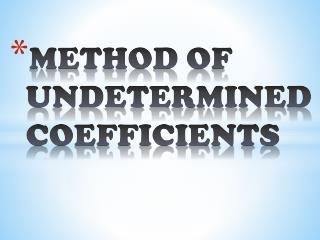# METHOD OF UNDETERMINED COEFFICIENTS - PowerPoint PPT PresentationDownload PresentationMETHOD OF UNDETERMINED COEFFICIENTS

METHOD OF UNDETERMINED COEFFICIENTSDownload Presentation## METHOD OF UNDETERMINED COEFFICIENTS

- - - - - - - - - - - - - - - - - - - - - - - - - - - E N D - - - - - - - - - - - - - - - - - - - - - - - - - - -
##### Presentation Transcript

1. METHOD OF UNDETERMINED COEFFICIENTS

2. Example

3. TRY THIS ALSO….. 1 2 3

4. METHOD OF VARIATION OF PARAMETER

5. METHOD OF SOLUTION Identify a and f(x) Substitute to general solution: Determine y1 and y2 (obtain from general solution of homogeneous equation y=Ay1+ By2) Evaluate u and v Evaluate Wronskian,

6. Example

7. EULER EQUATION

8. It called second order linear differential equation with variable coefficient • Euler equation: • Use substitution thus , and to reduce: • Solve it and get the answer in term of x and y From variable coefficient To constant coefficient

9. Solve it…… 1 2 3 4

10. APPLICATION-VIBRATING SPRING-

11. In equilibrium, [figure 1(b)] according to Newton's First Law, the resultant force is zero, So: • In this case, the spring stretched as far as s. according to Hooke's law • From 1 and 2 obtain • Next in equilibrium, the mass is pulled down a distance x and released. According to Hooke's law this spring elongation when F is: 1 2 3 4

12. According to Newton’s Second Law: • From equation 4, • When the resistance is negligible, the resistance equal to zero, so equation 5 becomes 5 6

13. From equation 6 or is called second order linear homogeneous differential equation: • From 7, get the general solution as 7 Equation of Motion

14. The period of free vibrations • Frequency is • If initial condition is given, find the value of A and B in equation of motion.

15. EXAMPLE

16. THE END…….